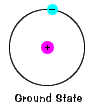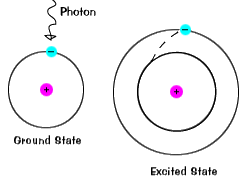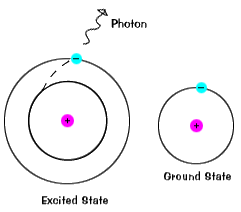# National Aeronautics and Space Administration

## Goddard Space Flight Center# X-ray Emission from Atoms

### Inside the Atom

An atom has a nucleus, which contains particles of positive charge (protons) and particles of neutral charge (neutrons). Surrounding the nucleus of an atom are shells of electrons, which are small negatively charged particles. Each shell has a specific energy associated with it. Within these shells, the electrons move around the nucleus of the atom.The ground state of an electron, the energy level it normally occupies, is the state of lowest energy for that electron. (Note that electron doesn't actually move about the nucleus in a circle. See The Quantum Story below.)When an electron occupies an energy shell greater than its ground state, it is in an excited state. An electron can become excited if it is given extra energy, such as when it absorbs a photon or if collides with a nearby atom or particle.An electron does not stay in an excited state for very long - it soon returns to the ground states. When it does so, a photon is emitted that has the same energy as the difference in the energy level between the excited state and the ground state.

So an electron moving from one energy shell to a lower one emits a photon of a specific energy. Since the energy and wavelength of the photon are related, we see this photon at a specfic wavelength in the spectrum. We refer to these as a "line" in the spectrum. Because there are many energy shells in any particular atom, there are many different possible energies with different initial and final values. When an atom is in an excited state, the electron can drop all the way to the ground state, or stop in an intermediate level.

Below is the optical spectrum for hydrogen. The distinct lines near 435 nm, 487 nm, and 655 nm show transitions from the 5th, 4th and 3rd energy shells, respectively, into the 2nd energy shell.

A hydrogen atom has one proton and one electron. That makes it easy to understand, and scientists can calculate exactly what energy the electron has in each shell. But hydrogen is also the least energetic element. Even the most energetic line hydrogen emits (when an electron drops down from the second shell to the first) has only enough energy to be an ultraviolet photon. So hydrogen atoms do not emit X rays.

So what elements emit X-ray lines? The more protons an element has, more energetic its lines can be. Carbon atoms (6 protons each) can emit X rays. But carbon lines are at the low end of X rays. Many X-ray instruments cannot detect these photons. Of the common elements in the universe, iron (26 protons) and oxygen (8 protons) usually are the two most prominent sources of X-ray lines.

### The Quantum Story

When first learning about the structure of atoms, it is common to hear about the electrons being in "orbitals" that have different energies. We then picture the electron being in a specific location in that orbit. This is convenient, but not the most accurate way to model the electrons in an atom.

Although each shell does have a precise energy, the position of the electron is envisioned as being smeared out into an "electron cloud" surrounding the nucleus. So, in our quantum story, we don't know the precise location of the electron, only where it is likely to be.

So instead of electrons moving about the nucleus in a plane (like the planets in the solar system) electrons move about the nucleus in three-dimensions. Further, the electron cloud of each energy level of an atom has a different shape. There are mathematical equations that will tell you the probability of the electron's location within that shell.

Let's consider the hydrogen atom.Probable locations of the electron in the ground state of the hydrogen atom.

At the left is a graph of the probable location of the electron in the ground state. The nucleus is at the center, and where the graph is brightest is where the electron is most likely to be located. What you see here is a cross section. That is, you have to imagine the picture rotated around the vertical axis. So the region inhabited by this electron looks like a disk in this graph, but it is actually a sphere.Probable locations of the electron in an excited state of hydrogen

To the right is an atom of hydrogen in an excited state. Notice that at the center, where the nucleus is, the picture is dark, indicating that the electron is unlikely to be there. The two bright regions, where the electron is most likely to be found, are really just one region. Remember, you have to mentally rotate this around a vertical axis, so that in three dimensions the light region is really doughnut-shaped.